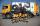Bags

The first bag has a weight of 18 kg, the second bag is a one-third lighter than the first and the third bag has three times less the weight of all three bags together. Determine their weights.

Result

a =  18 kg
b =  12 kg
c =  15 kg

Solution:c = (18+12+c)/3

2c = 30

c = 15

Calculated by our simple equation calculator.

Leave us a comment of example and its solution (i.e. if it is still somewhat unclear...):Be the first to comment!To solve this verbal math problem are needed these knowledge from mathematics:

Do you have a linear equation or system of equations and looking for its solution? Or do you have quadratic equation?

Next similar examples:

1. Two numbersWe have two numbers. Their sum is 140. One-fifth of the first number is equal to half the second number. Determine those unknown numbers.
2. Father and sonFather and son weigh together 108kg. The father weighs 2.6 times more than his son. How much does weight my father and son count?
3. SummerjobThree students participated in the summerjob. Altogether they earn 1780, -. Peter got a third less than John and Paul got 100,- more than Peter. How much got every one of them?
4. Three brothersThe three brothers have a total of 42 years. Jan is five years younger than Peter and Peter is 2 years younger than Michael. How many years has each of them?
5. Collecting dayAt collecting day at school pupils of three classes took total of 1063 kg of paper. Class B collected half more than A Class and class C 55 kg more than B. How many kg of paper collected each class?
6. TheatroTheatrical performance was attended by 480 spectators. Women were in the audience 40 more than men and children 60 less than half of adult spectators. How many men, women and children attended a theater performance?
7. Vegan shopTo the shops brought together 23.2 kg of goods, fruits, vegetables and nuts. fruit was 4.7 kg more than vegetables, nuts was 1.5 kg less than the fruit. Determine the amount of fruits, vegetables and nuts.
8. Fifth of the numberThe fifth of the number is by 24 less than that number. What is the number?
9. ChildrenThe group has 42 children. There are 4 more boys than girls. How many boys and girls are in the group?
10. CandiesIf Alena give Lenka 3 candy will still have 1 more candy. If Lenka give Alena 1 candy Alena will hame twice more than Lenka. How many candies have each of them?
11. Hotel roomsIn the 45 rooms, there were 169 guests, some rooms were three-bedrooms and some five-bedrooms. How many rooms were?
12. EquationsSolve following system of equations: 6(x+7)+4(y-5)=12 2(x+y)-3(-2x+4y)=-44
13. Linsys2Solve two equations with two unknowns: 400x+120y=147.2 350x+200y=144
14. ATC campThe owner of the campsite offers 79 places in 22 cabins. How many of them are triple and quadruple?
15. Rabbits 3Viju has 40 chickens and rabbits. If in all there are 90 legs. How many rabbits are there with Viju?
16. Football match 4In a football match with the Italy lost 3 goals with Germans. Totally fell 5 goals in the match. Determine the number of goals of Italy and Germany.
17. TreesAlong the road were planted 250 trees of two types. Cherry for 60 CZK apiece and apple 50 CZK apiece. The entire plantation cost 12,800 CZK. How many was cherries and apples?